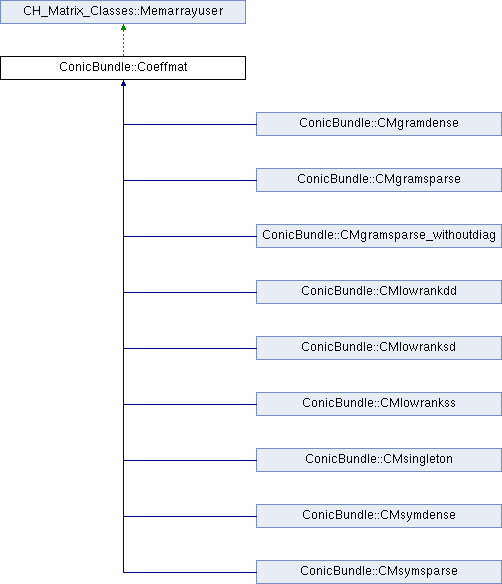ConicBundle
ConicBundle::Coeffmat Class Referenceabstract

defines a base class for coefficient matrices in semidefinite programming, in particular for use with MatrixSDPfunction, see implemention of a PSCOracle (PSCAffineFunction). More...

`#include <Coeffmat.hxx>`

Inheritance diagram for ConicBundle::Coeffmat:## Public Member Functions

Coeffmat (bool del_by_CoeffmatPointer=true)
default constructor; set del_by_CoeffmatPointer==true if a CoeffmatPointer reducing the use_cnt to zero should delete this

void set_deletion_by_CoeffmatPointer (bool dbMP)
if set to true any CoeffmatPointer that reduces use_cnt to zero will delete this object

virtual Coeffmattype get_type () const
returns its Coeffmattype

virtual CoeffmatInfoget_info ()
returns the user information

virtual const CoeffmatInfoget_info () const
returns the user information in const form

virtual void set_info (CoeffmatInfo *cip)
deletes the old and sets new user information

virtual Coeffmatclone () const =0
makes an explicit copy of itself and returns a pointer to it

virtual CH_Matrix_Classes::Integer dim () const =0
returns the order of the represented symmetric matrix

virtual CH_Matrix_Classes::Real operator() (CH_Matrix_Classes::Integer i, CH_Matrix_Classes::Integer j) const =0
returns the value of the matrix element (i,j)

virtual void make_symmatrix (CH_Matrix_Classes::Symmatrix &S) const =0
returns a dense symmetric constraint matrix (useful for testing)

virtual CH_Matrix_Classes::Real norm (void) const =0
returns the Frobenius norm of the matrix

virtual Coeffmatsubspace (const CH_Matrix_Classes::Matrix &P) const =0
delivers a new object on the heap corresponding to the matrix P^TAP, the caller is responsible for deleting the object

virtual void multiply (CH_Matrix_Classes::Real d)=0
multiply constraint permanentely by d; this is to allow scaling or sign changes in the constraints

virtual CH_Matrix_Classes::Real ip (const CH_Matrix_Classes::Symmatrix &S) const =0
returns ip(*this,S)=trace(*this*S), the trace inner product

virtual CH_Matrix_Classes::Real gramip (const CH_Matrix_Classes::Matrix &P) const =0
returns ip(*this,PP^T)=trace P^T(*this)P

virtual CH_Matrix_Classes::Real gramip (const CH_Matrix_Classes::Matrix &P, CH_Matrix_Classes::Integer start_row, const CH_Matrix_Classes::Matrix *Lam=0) const =0
returns ip(*this,Q*Diag(Lam)*Q^T)=trace Q^T(*this)Q*Diag(Lam) for Q=P.rows(start_row,start_row+dim-1), where Diag(Lam) is the identity if not given

virtual void addmeto (CH_Matrix_Classes::Symmatrix &S, CH_Matrix_Classes::Real d=1.) const =0
computes S+=d*(*this);

virtual void addprodto (CH_Matrix_Classes::Matrix &A, const CH_Matrix_Classes::Matrix &B, CH_Matrix_Classes::Real d=1.) const =0
comutes A+=d*(*this)*B

virtual void addprodto (CH_Matrix_Classes::Matrix &A, const CH_Matrix_Classes::Sparsemat &B, CH_Matrix_Classes::Real d=1.) const =0
computes A+=d*(*this)*B

virtual void left_right_prod (const CH_Matrix_Classes::Matrix &P, const CH_Matrix_Classes::Matrix &Q, CH_Matrix_Classes::Matrix &R) const =0
computes R=P^T*(*this)*Q

virtual CH_Matrix_Classes::Integer prodvec_flops () const =0
returns an estimate of number of flops to compute addprodto for a vector

virtual int dense () const =0
returns 1 if its structure is as bad as its dense symmetric representation, otherwise 0

virtual int sparse () const =0
returns 0 if not sparse, otherwise 1

virtual int sparse (CH_Matrix_Classes::Indexmatrix &I, CH_Matrix_Classes::Indexmatrix &J, CH_Matrix_Classes::Matrix &val, CH_Matrix_Classes::Real d=1.) const =0
returns 0 if not sparse. If it is sparse it returns 1 and the nonzero structure in I,J and val, where val is multiplied by d. Only the upper triangle (including diagonal) is delivered

virtual int support_in (const CH_Matrix_Classes::Sparsesym &A) const =0
returns 0 if the support of the costraint matrix is not contained in the support of the sparse symmetric matrix A, 1 if it is contained.

virtual CH_Matrix_Classes::Real ip (const CH_Matrix_Classes::Sparsesym &A) const =0
returns the inner product of the constraint matrix with A

virtual void project (CH_Matrix_Classes::Symmatrix &S, const CH_Matrix_Classes::Matrix &P) const =0
computes S=P^T*(*this)*P

virtual void add_projection (CH_Matrix_Classes::Symmatrix &S, const CH_Matrix_Classes::Matrix &P, CH_Matrix_Classes::Real alpha=1., CH_Matrix_Classes::Integer start_row=0) const =0
computes S+=alpha*Q^T(*this)Q for Q=P.rows(start_row,start_row+dim-1)

virtual const CH_Matrix_Classes::Matrixpostgenmult (const CH_Matrix_Classes::Matrix &B, CH_Matrix_Classes::Matrix &C, CH_Matrix_Classes::Real alpha=1., CH_Matrix_Classes::Real beta=0., int btrans=0) const =0
computes C= alpha*(*this)*B^(T if btrans) + beta*C, C is also returned

virtual const CH_Matrix_Classes::Matrixpregenmult (const CH_Matrix_Classes::Matrix &B, CH_Matrix_Classes::Matrix &C, CH_Matrix_Classes::Real alpha=1., CH_Matrix_Classes::Real beta=0., int btrans=0) const =0
computes C= alpha*B^(T if btrans)*(*this) + beta*C, C is also returned

virtual int equal (const Coeffmat *p, double tol=1e-6) const =0
returns 1, if p is the same derived class and entries differ by less than tol, otherwise zero

virtual std::ostream & display (std::ostream &o) const =0
display constraint information

virtual std::ostream & out (std::ostream &o) const =0
put entire contents onto outstream with the class type in the beginning so that the derived class can be recognized by in().

virtual std::istream & in (std::istream &i)=0
counterpart to out(), does not read the class type, though. This is assumed to have been read in order to generate the correct class

## Protected Attributes

Coeffmattype CM_type
in order to enable type identification

CoeffmatInfoinfop
allows the user to specify and output additional information

## Private Attributes

CH_Matrix_Classes::Integer use_cnt
Each CoeffmatPointer pointing to his increase the use_cnt by one and it reduces it by one once it stops pointing to it; if use_cnt turns zero and deletion_by_CoeffmatPointer is true, the object will be deleted by the respective CoeffmatPointer.

bool deletion_by_CoeffmatPointer
set deletion_by_CoeffmatPointer==true if a CoeffmatPointer reducing the use_cnt to zero should delete this

## Friends

class CoeffmatPointerProtected Member Functions inherited from CH_Matrix_Classes::Memarrayuser
Memarrayuser ()
if memarray is NULL, then a new Memarray is generated. In any case the number of users of the Memarray is incremented

virtual ~Memarrayuser ()
the number of users is decremented and the Memarray memory manager is destructed, if the number is zero.Static Protected Attributes inherited from CH_Matrix_Classes::Memarrayuser
static Memarraymemarray
pointer to common memory manager for all Memarrayusers, instantiated in memarray.cxx

## Detailed Description

defines a base class for coefficient matrices in semidefinite programming, in particular for use with MatrixSDPfunction, see implemention of a PSCOracle (PSCAffineFunction).

The class supports several different needs, e.g. it should work well with the requirements of MaxEigOracle as well as those of the eigenvalue computations with Bigmatrix. Furthermore there is support for writing and reading the various implementations.

The documentation for this class was generated from the following file: